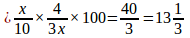# Target SBI PO  Mains – Aptitude  Day 2 – Percentage

Q.1) How much percent of an article’s marked price be reduced so as it can be sold at a rate of 7.5% profit if the marked price be Rs.20 more than the cost price?

a) 10.41%

b) 9.08%

c) 8.11%

d) Cannot be determined

e) None of these

d) Cannot be determined

Since the marked price of the article is not given, we cannot determine the required percentage.

Q.2) If person A’s salary is 20% more than another person B’s salary, then how much percentage less is B getting the salary?

a) 33.33%

b) 16.67%

c) 24.24%

d) 12.12%

e) None of these

b) 16.67%

As the first person earns 20% more than the second person,

Required percentage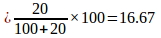Q.3) How much percent can Joe increases the consumption of petrol if the price per litre has been dropped to Rs.64.35 to Rs.61.55?

a) 4.54%

b) 4.35%

c) 4.24%

d) 4.17%

e) 4.62%

a) 4.54%

The difference between the price= Rs.2.8 which is 4.35% of Rs.64.35.

Required percentage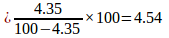Q.4) If the number of cars manufactured by a company on the first day of July is 24000. If the number of cars is increased to 81000 in the end of September, then pick the correct option.

a) The rate of production of the cars is increased by 50% every month

b) The rate of production of the cars is increased by 25% every month

c) The rate of production of the cars is increased by 75% every month

d) Cannot be determined

e) None of these

a) The rate of production of the cars is increased by 50% every month

At the end of July, the count of cars=24000+50% of 24000=36000

At the end of august, the count of cars=36000+50% of 36000=54000

At the end of September, the count of cars=54000+50% of 54000=81000

Q.5) Sandy earns Rs.4, 80, 000 per annum. In a month, she spends 15% of her monthly salary for rent, 20% on groceries and 30% on savings. She gives the remaining money to her son and daughter in the ratio of 3:4 for their term fees. What is the percentage of money given to her daughter’s fees per annum?

a) 24%

b) 32%

c) 20%

d) 16%

e) None of these

c) 20%

Monthly income= Rs.40000

Apart from all the expenses, she has given 35% of the money to their children. So, 35% of 40000=14000

The money given to her daughter is 8000 which is 20% of her monthly salary.

Q.6) Luther sold a lamp at 5% loss and another lamp Rs.541.5. He accrues an overall loss of 5%. What is the cost price of two lamps where the selling price of two lamps is equal?

a) 1440

b) 1340

c) 1240

d) 1140

e) None of these

d) 1140

Let the cost price of a lamp be x.

Given that,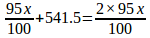X=Rs.570

Q.7) A man buys 12 bats for Rs.4500 and sells 8 bats for Rs.3200. Find the percentage of profit for a couple of bats?

a)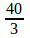b)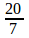c)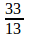d)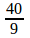e) None of these

e) None of these

Cost price of a bat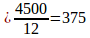Selling price of a bat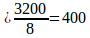Profit percentage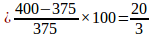Q.8) Which of the following is a better offer?

I. Three successive discounts of 12%, 15% and 5%

II. Three successive discounts of 10%, 10% and 12%

a) First one is better offer

b) Second one is better offer

c) Both are equal

d) Cannot be determined

e) None of these

a) First one is better offer

Let us take the cost price of article be X.

For the first case,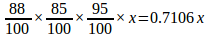For the second case,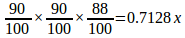Obviously, first offer is better.

Q.9) If a seller makes a successive discounts of 6%, 10% and 14% on a product of marked price Rs.2000. What is the cost price if he incurs a profit of 1%?

a) 1425

b) 1430

c) 1440

d) 1415

e) None of these

c) 1440

Let the cost price be x.

After successive discounts, the selling price be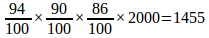He makes a profit of 1%,

So,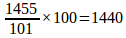Q.10) If a discount of 10% is given on the marked price of a radio, the seller gets 20% profit. If the discount rate is increased to 15%, then the gain percent is?

a)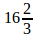b)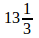c)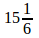d) Cannot be determined

e) None of these

b)

Let the marked price be x.

If 10% discount is given, then the selling price would be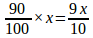Now, selling price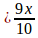Gain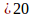CP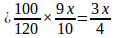Again, SP=85% of Rs. x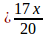Gain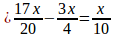Gain%